# Graphing Sine, Cosine And Tangent Worksheet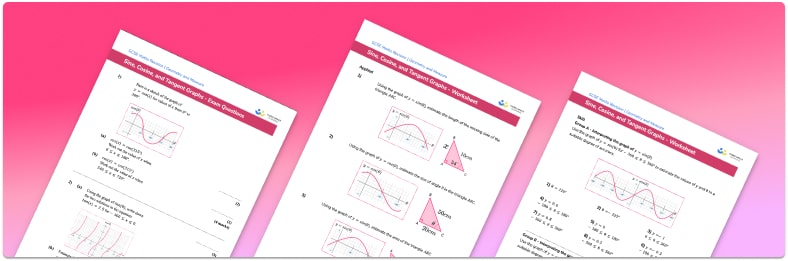• Section 1 of the graphing sine, cosine and tangent worksheet contains 27 skills-based graphing sine, cosine and tangent questions, in 3 groups to support differentiation
• Section 2 contains 3 applied graphing sine, cosine and tangent questions with a mix of worded problems and deeper problem solving questions
• Section 3 contains 3 foundation and higher level GCSE exam style graphing sine, cosine and tangent questions
• Answers and a mark scheme for all graphing sine, cosine and tangent questions are provided
• Questions follow variation theory with plenty of opportunities for students to work independently at their own level
• All questions created by fully qualified expert secondary maths teachers
• Suitable for GCSE maths revision for AQA, OCR and Edexcel exam boards

• This field is for validation purposes and should be left unchanged.

You can unsubscribe at any time (each email we send will contain an easy way to unsubscribe). To find out more about how we use your data, see our privacy policy.

### Graphing sine, cosine and tangent at a glance

By entering different values of x into the equations y=sin(x), y=cos(x) and y=tan(x), we can plot the graphs of the trig functions sine, cosine and tangent. The graphs can also be created using a unit circle.

We often plot the graphs for values of x from 0o to 360o but we can extend them to show any positive or negative angle.

There are some special features of graphs of trigonometric functions. The graphs of sine and cosine both have an amplitude of 1 and period of 360o. The graph of the tangent function is different from the others and has a period of 180o and also an asymptote every 180o.

Once we have plotted the graphs of the sine function, cosine function and tangent function, we can use these to find solutions, or approximate solutions, to equations involving sin x, cos x or tan x.

Transformations of graphs can also be applied to sine graphs, cosine graphs and tangent graphs. They can undergo stretches, horizontal and vertical shifts and reflections in the same way that any other graph can.

Looking forward, students can then progress to additional geometry worksheets, for example a 3D shapes worksheet or .For more teaching and learning support on Geometry our GCSE maths lessons provide step by step support for all GCSE maths concepts.

## Related worksheets

Exact Trig Values Worksheet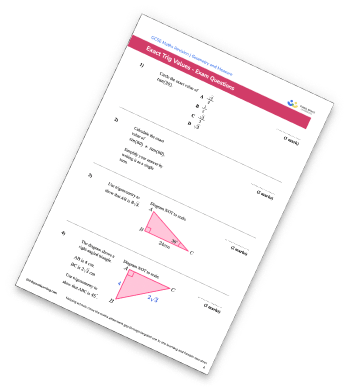Hypotenuse Worksheet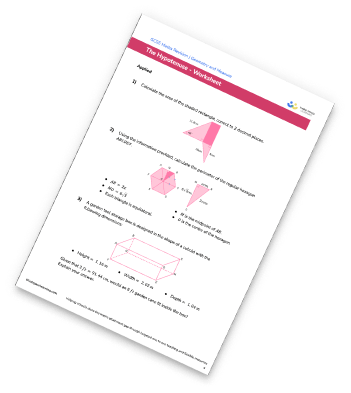Trigonometry Worksheet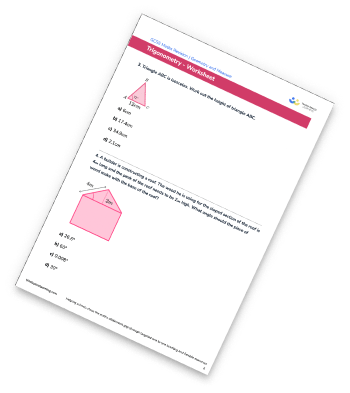Sine Rule Worksheet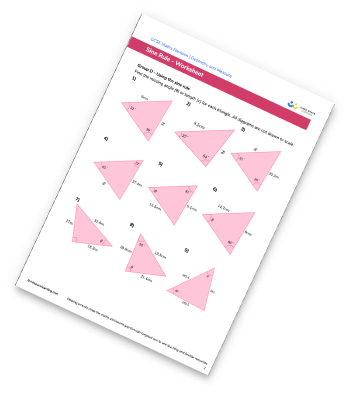## Do you have KS4 students who need more focused attention to succeed at GCSE?There will be students in your class who require individual attention to help them succeed in their maths GCSEs. In a class of 30, it’s not always easy to provide.

Help your students feel confident with exam-style questions and the strategies they’ll need to answer them correctly with our dedicated GCSE maths revision programme.

Lessons are selected to provide support where each student needs it most, and specially-trained GCSE maths tutors adapt the pitch and pace of each lesson. This ensures a personalised revision programme that raises grades and boosts confidence.

Find out more